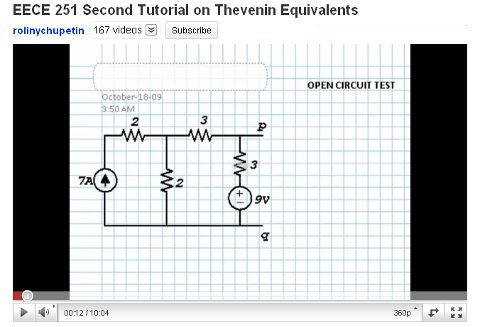# Second Tutorial on Thevenin EquivalentsThis is Thevenin equivalents video tutorial part 2. This video shows another way to calculate Thevenin equivalents on the open circuit. In this video the Thevenin equivalents is calculated using two current loop. From first loop, the result of V1 is 12.75V. Voc can be calculated using V1, and the result is 10.88V. Besides that, in this video also shown how to calculated Thevenin equivalents using short circuit method.